### We're updating the default styles for Matplotlib 2.0

Learn what to expect in the new updates#### Previous topic

pylab_examples example code: barcode_demo.py

#### Next topic

pylab_examples example code: boxplot_demo2.py

# pylab_examples example code: boxplot_demo.py¶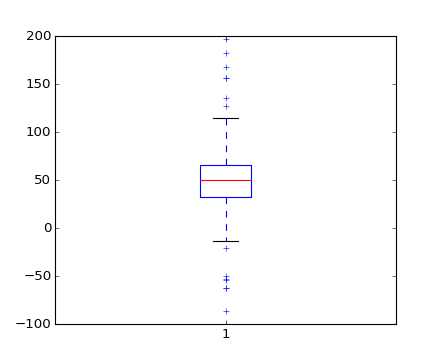(png, hires.png, pdf)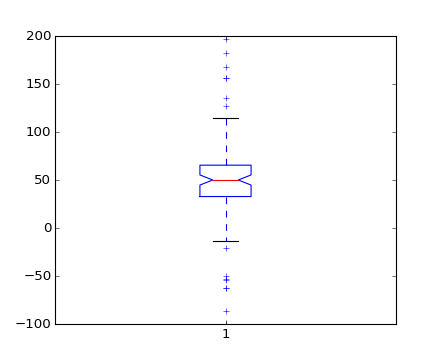(png, hires.png, pdf)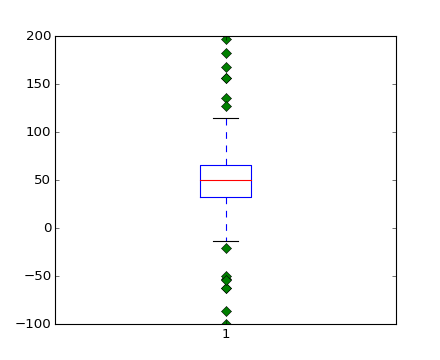(png, hires.png, pdf)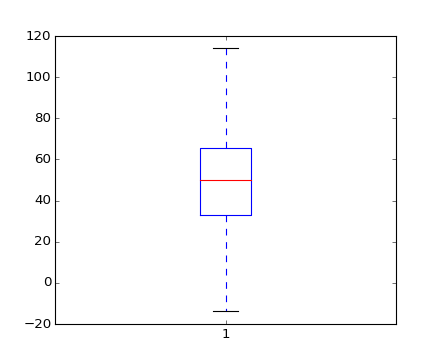(png, hires.png, pdf)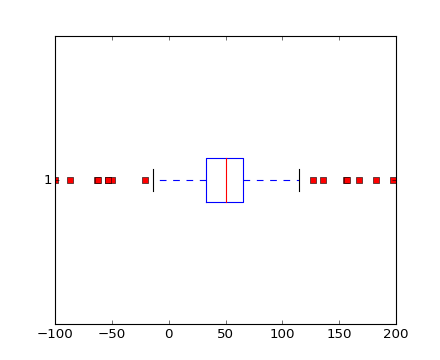(png, hires.png, pdf)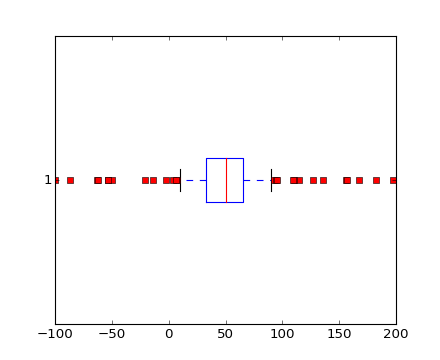(png, hires.png, pdf)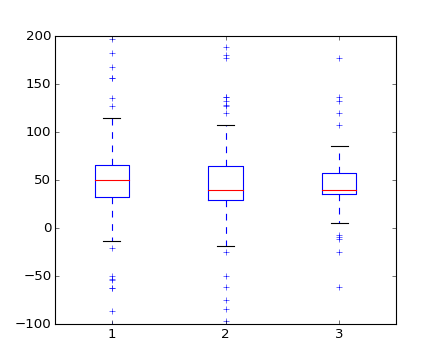(png, hires.png, pdf)

```#!/usr/bin/python

#
# Example boxplot code
#

from pylab import *

# fake up some data
center = ones(25) * 50
flier_high = rand(10) * 100 + 100
flier_low = rand(10) * -100
data =concatenate((spread, center, flier_high, flier_low), 0)

# basic plot
boxplot(data)

# notched plot
figure()
boxplot(data,1)

# change outlier point symbols
figure()
boxplot(data,0,'gD')

# don't show outlier points
figure()
boxplot(data,0,'')

# horizontal boxes
figure()
boxplot(data,0,'rs',0)

# change whisker length
figure()
boxplot(data,0,'rs',0,0.75)

# fake up some more data
center = ones(25) * 40
flier_high = rand(10) * 100 + 100
flier_low = rand(10) * -100
d2 = concatenate( (spread, center, flier_high, flier_low), 0 )
data.shape = (-1, 1)
d2.shape = (-1, 1)
#data = concatenate( (data, d2), 1 )
# Making a 2-D array only works if all the columns are the
# same length.  If they are not, then use a list instead.
# This is actually more efficient because boxplot converts
# a 2-D array into a list of vectors internally anyway.
data = [data, d2, d2[::2,0]]
# multiple box plots on one figure
figure()
boxplot(data)

show()
```

Keywords: python, matplotlib, pylab, example, codex (see Search examples)